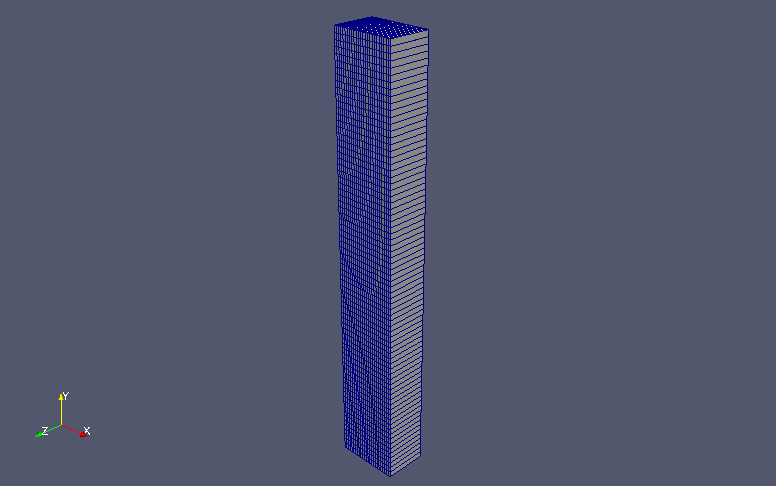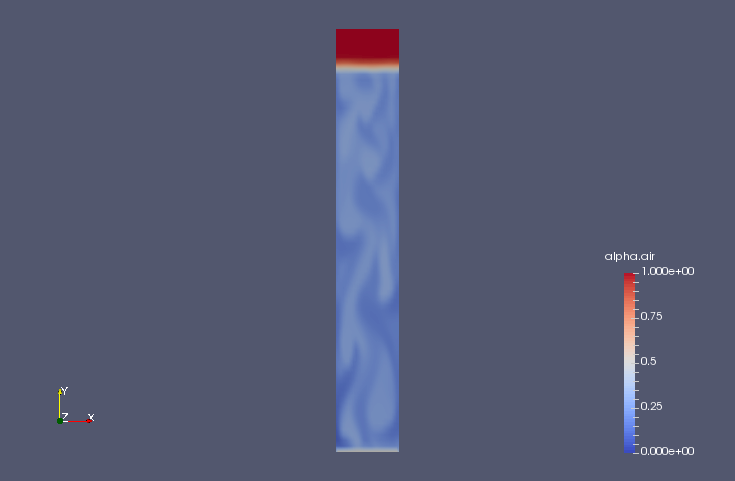﻿ Blowing air into water column (LES) - XSim

# Blowing air into water column (LES)

Update: July 28, 2017
OpenFOAM 4.x

## Case directory

\$FOAM_TUTORIALS/multiphase/twoPhaseEulerFoam/LES/bubbleColumn

## SummaryModel geometry

We calculate the mixing of air into the water column for 100 seconds. Air flows in from the region "inlet" at the bottom of the water column at (0, 0.1, 0) m/s and out from the region "outlet". We assume that the ratio of water to air is 1:1 in the region "inlet", and the calculation is performed as a two-dimensional problem with one mesh in the z-direction.

The properties of the air and water are set in the files "constant/phaseProperties", "constant/thermophysicalProperties.air", and "constant/thermophysicalProperties.water".

The turbulence models for each fluid are set in the files "constant/turbulenceProperties.air" and "constant/turbulenceProperties.water" as follows.

```simulationType  LES;
LES
{
turbulence      on;
printCoeffs     on;
delta           cubeRootVol;
cubeRootVolCoeffs
{
}
}
```
LES settings for the air
```simulationType  LES;
LES
{
LESModel NicenoKEqn; //SmagorinskyZhang;
turbulence      on;
printCoeffs     on;
delta           cubeRootVol;
cubeRootVolCoeffs
{
}
}
```
LES settings for the water

The meshes are as follows, and the number of mesh is 1875.Meshes

The calculation result is as follows.

Volume ratio of air (alpha.air)Volume ratio of air at final time (alpha.air)

## Commands

cp -r \$FOAM_TUTORIALS/multiphase/twoPhaseEulerFoam/LES/bubbleColumn bubbleColumn
cd bubbleColumn

blockMesh

setFields
twoPhaseEulerFoam

paraFoam

## Calculation time

15 minutes 41.35 seconds *Single, Inter(R) Core(TM) i7-2600 CPU @ 3.40GHz 3.40GHz